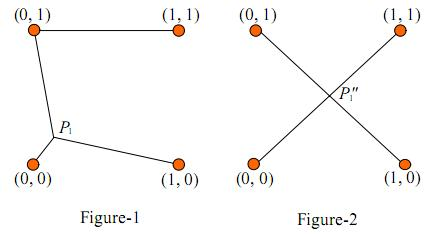favorite Saytın davamlılığını təmin etmək üçün sizin kəməyinizə ehtiyacımız vardır, ətrafli məlumat üçün bannerə klikləyin
Məsələlər

# Square

Given a square at [0, 1]×[0, 1] that has N points ( P1, P2, ..., PN) in the square (you may assume that different points can be at the same position), we can connect the N points and the four corners of the square with some line segments so that through these segments any two of the N+4 points can reach each other (directly or indirectly). The graph length is defined as the total length of the line segments. When N points’ positions are fixed, there must exist a way of connecting them, such that it will make the shortest graph length. We can use LEN (P1, P2, ..., PN) to record the graph length using this way of connecting.

In this situation, LEN (P1, P2, ..., PN) is a function of P1, P2, ..., PN. When P1, P2, ..., PN change their positions, LEN (P1, P2, ..., PN) also changes. It's easy to prove that there exist some P1', P2', ..., PN' in the square such that LEN (P1', P2', ..., PN') is at its minimum.

Given the initial positions of N points, your task is to find out N points P1'', P2'', ..., PN'' in the square such that |P1P1''| + |P2P2''| + ... + |PNPN''| is minimum and LEN (P1'', P2'', ..., PN'') = LEN (P1', P2', ..., PN'). You are requested to output the value of |P1P1''| + |P2P2''| + ... + |PNPN''|, where |PiPi''| is the distance between Pi and PiPi''.For example, Figure-1 gives the initial position of P1 and the way of connecting to obtain LEN (P1). In Figure-2, it gives the position of P1'', which is at the center of the square, and the way of connecting to obtain LEN (P1''). It can be proved that LEN (P1'') = LEN (P1'); your job is to output the distance between P1 and P1''.

Input

The input consists of several test cases. For each test case, the first line consists of one integer N (1N100), the number of points, and N lines follow to give the coordinates for every point in the following format:

x y

Here, x and y are float numbers within the value [0, 1].

A test case of N = 0 indicates the end of input, and should not be processed.

Output

For each test case, output the value of |P1P1''| + |P2P2''| + ... + |PNPN''|. The value should be rounded to three digits after the decimal point.

Zaman məhdudiyyəti 1 saniyə
```1
```0.300